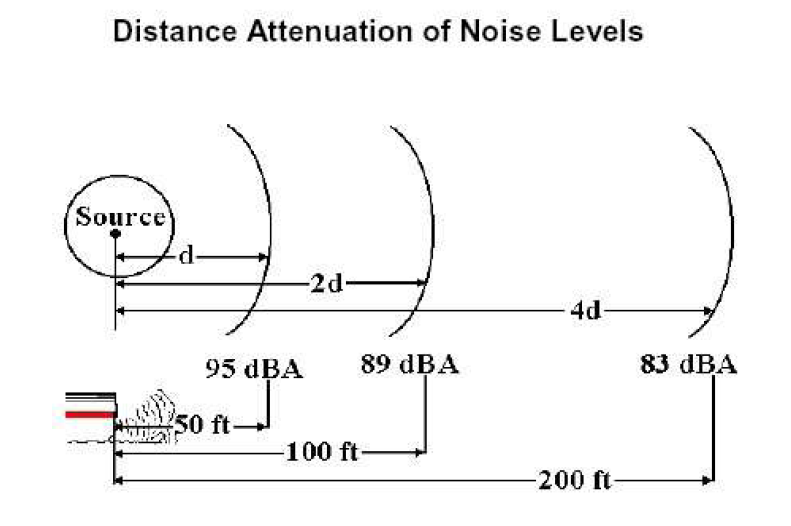Miscellaneous

# Sound pressure level and sound power level

All machines generate sound when they are in operation. The propagated sound waves cause small changes in the ambient air pressure while traveling. A sound source produces sound power and this generates a sound pressure fluctuation in the air. Sound power is the cause of this, whereas sound pressure is the effect.

To put it more simply, what we hear is sound pressure, but this sound pressure is caused by the sound power of the emitting sound source. To make a comparison, imagine for example a simple light bulb. The bulb's power wattage (in W) represents the sound power, whereas the bulb's light intensity represents the sound pressure.

Sound power (Lw) is calculated in W. The sound power level is expressed in decibel (dB) with respect to a standardized reference value:

Lw = 10×log (W/Wo).... (1), where:

Lw = sound power level (in dB)
W = actual sound power (in W)
Wo = reference sound power (10-12 W): The normal reference level is 10-12 W, which is the lowest sound persons of excellent hearing can recognize.

Sound pressure (Lp) is expressed in Pa. Sound pressure level is also expressed in decibels (dB) and is also correlated to a standardized reference value:

Lp = 20×log (P/Po).... (2), where:

Lp = sound pressure level (in dB)
P = actual sound pressure (in Pa)
Po = reference sound pressure (20×10-6 Pa): The threshold of audibility, or the minimum pressure fluctuation that can be detected by the human ear is approximately 20×10-6 Pa at 1000 Hz.

Sound pressure level is typically measured with portable devices called decibelometers.

### Comparison between sound power level and sound pressure level

Sound power does not generally depend on the environment. On the contrary, the sound pressure depends on the distance from the source and also on the acoustic environment where the sound wave is produced.

For example, in case of indoor installations, sound pressure depends on the size of the room and on the sound absorption capacity of the surfaces. For example, in case the room walls don't absorb all the sound but reflect parts of it, then the sound pressure will increase due to the so called reverberation effect. (reverberation time is broadly defined as the time it takes for the sound pressure to reduce by 60 dB after the sound emitting source has been shut off).

As far as the size of the room is concerned, a bigger room surface area will necessitate a bigger sound pressure level, which of course will not be proportional to the increase of the room size surface increase.

### Relation between sound pressure level and sound power level

Assuming sound is emitted from a point-like source, either inside a room without any hard reflecting walls or outside, where no obstructions (e.g. walls) are close to the sound source, the sound is distributed equally towards all directions (sound intensity is constant at all points across a spherical surface around the noise source, therefore sound pressure can be considered proportional to the spherical radius/diameter of this surface).

When the distance from the source is doubled, the spherical surface will have quadrupled (i.e. it will have become 4 times bigger, considering that A=π×D2).

Taking into consideration equation (2), it is concluded that the sound pressure level decreases by 6 dB every time the distance from the sound source is doubled, since assuming we speak about position 1 and position 2, we get that:

Lp2 = Lp1 + 20×log (R1) - 20×log(R2)

and assuming that R1= x m, whereas R2 = 2x m (double the value of R1), it is concluded that:

Lp2 = Lp1 - 20×log2 --> Lp2 = Lp1 -20×0.30103 --> Lp2 = Lp1 - 6.02 (in dB)

This is illustrated more clearly at Figure 1.Figure 1 - Distance attenuation of noise levels

Considering that all above-mentioned assumptions are valid, then we have as an approximation the following equation:

Lp = Lw + 10×log(Q/4×π×r2) (3), where:

Lp = sound pressure level (in dB)
Lw = sound power level (in dB)
Q = direction factor (unitless)
r = distance to the sound source

Direction factor Q takes in general empirical values, typically ranging from 1 (if the sound source is located in the middle of the room) to 8 (if the sound source is located close to the intersection of three walls)

### Multiple Sound Sources

Adding two sound sources can't be done algebraically, since decibel values are logarithmic values. Consequently, if we add two identical sound sources of 60 dB, the resulting sound level will be 63 dB.
Two identical sound sources produce a 3 dB increase in sound power level.

Two identical sound sources produce a 3 dB increase in sound pressure level, assuming no other interference.